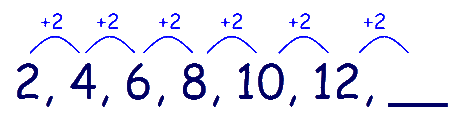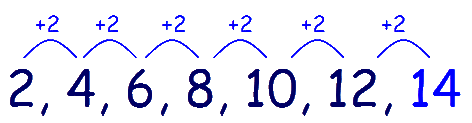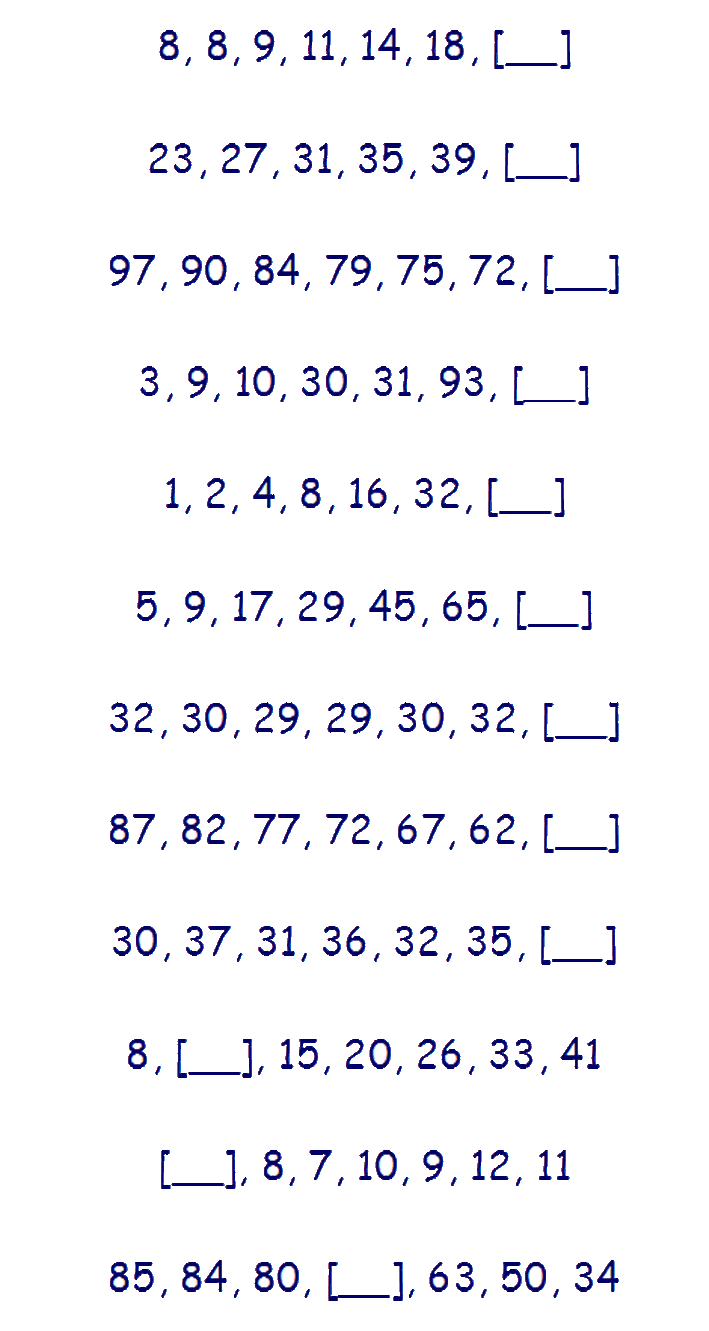# Type P - Number Series

With this type of question you are given a list of numbers that form a series with a logical sequence. One of the numbers in the series is missing.

You are required to find the missing number by working out the rule that the sequence of numbers is using. Then use that rule to extend the sequence to find the missing number.

### It is important that your child understands the concept of sequences before attempting this. Work through Number Sequences and Patterns first.

Lets look at an example:

# 2, 4, 6, 8, 10, 12, __

A useful strategy for dealing with sequences is to write them down, giving enough space to draw and label the hops from one term to another.Once the individual hops are labelled, it should be much easier to see the relationship between each number and discover the rule, in this case '+2,+2,+2,+2,+2 etc'. It is now a simple matter of extending the sequence using the rule '+2'. 12 + 2 =14.There are several types of sequences that can be used. The pattern can increase or
decrease, you may need to add, subtract, multiply or divide and the pattern
may change between each number. These are explained in
Number Sequences and Patterns

### Verbal Reasoning Type P - 'Number Series' practice